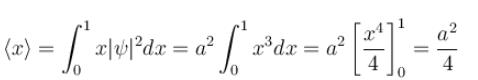Courses

# Postulates Of Quantum Mechanics MCQ Level - 1

## 10 Questions MCQ Test Modern Physics for IIT JAM | Postulates Of Quantum Mechanics MCQ Level - 1

Description
This mock test of Postulates Of Quantum Mechanics MCQ Level - 1 for Physics helps you for every Physics entrance exam. This contains 10 Multiple Choice Questions for Physics Postulates Of Quantum Mechanics MCQ Level - 1 (mcq) to study with solutions a complete question bank. The solved questions answers in this Postulates Of Quantum Mechanics MCQ Level - 1 quiz give you a good mix of easy questions and tough questions. Physics students definitely take this Postulates Of Quantum Mechanics MCQ Level - 1 exercise for a better result in the exam. You can find other Postulates Of Quantum Mechanics MCQ Level - 1 extra questions, long questions & short questions for Physics on EduRev as well by searching above.
QUESTION: 1

### A certain excited state of hydrogen atom is known to have a life of 2.5 × 10–14 s. The minimum error, with which the energy of the excited state can be measured is.

Solution:

The energy time uncertainty relation is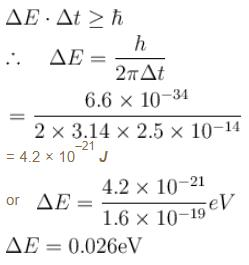The correct answer is: 0.026 eV

QUESTION: 2

### Which of the following is the eigenfunction of the linear momentum operator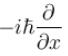with a positive eigenvalue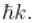Solution: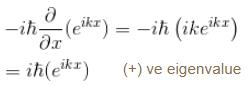Compare this with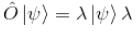is the eigenvalue

QUESTION: 3

### An eigenfunction of an operator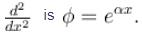The corresponding eigenvalue is.

Solution: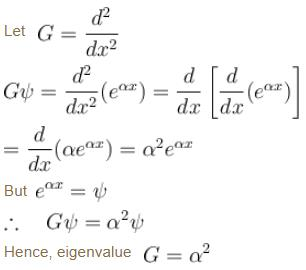QUESTION: 4

The ground state energy of an electron confined to a box 1Å wide is.

Solution:

a = 1 * 10-10m  m = 9.1 * 10-31kg

Energy of electron in ground state

E1 = h2/8ma2

⇒ [(6.625 * 10-34)2]/(8 * 9.1 * 10-31 * 1 * 10-10)

E1 = 6.016 * 10-18 J

QUESTION: 5

Which of the following values of A will properly normalize the wave function of a rigid dumbbell rotating about its centre having φ dependence as ψ(φ) = Aeimφ  where m is a quantum number.

Solution:

For normalization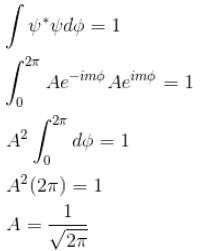The correct answer is: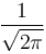QUESTION: 6

A particle of mass m has the wave function ψ(x, t) = eiωt (α cos kx + β sin kx) where α, β are complex constants and ω and k are real constants. The probability current density is equal to :

Solution:

Probability current density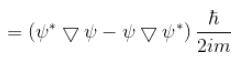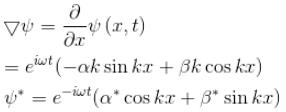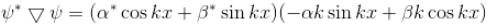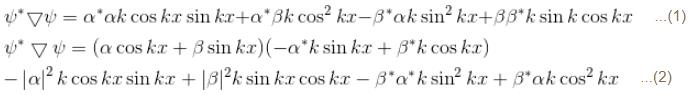subtract (2) and (1)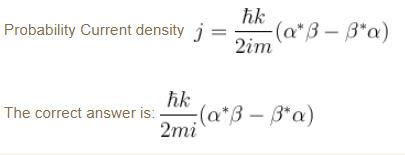QUESTION: 7

If de-Broglie wavelength of an electron is 73Å. Then the velocity of electron is. Given, me = 9.1 × 10–31 kg and h = 6.6 × 10–34 Js.

Solution: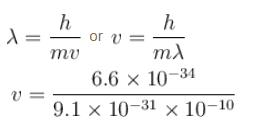= 9.93 × 104 m/s
The correct answer is: 9.93 × 104 m/s

QUESTION: 8

Which of the following is correct set of eigenvalues of the Hermitian matrix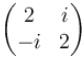Solution: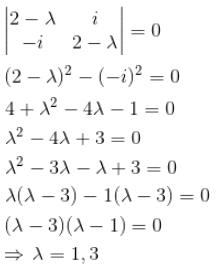The correct answer is: 1, 3

QUESTION: 9

If ψ is a normalized solution of the Schrödinger equation and Q is the operator corresponding to a physical quantity x, then the quantity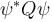may be integrated in order to obtain :

Solution:

The correct answer is: mean value of x

QUESTION: 10

A particle limited to the x–axis has the wave frequency ψ = ax between x = 0 and x = 1, ψ = 0 elsewhere. The expectation value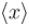of the particle's position is.

Solution: